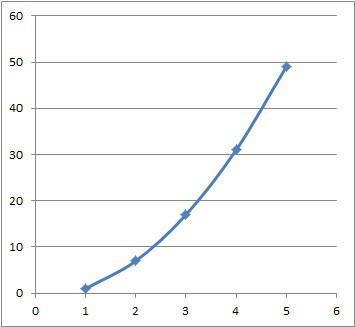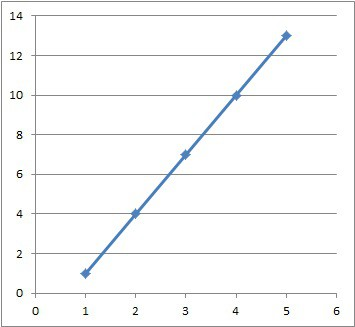# What Is A Quadratic Sequence? (3 Things To Know)

When working with sequences of numbers, it helps to be able to recognize patterns.  Some of them are arithmetic or geometric, and some are linear or quadratic.

So, what is a quadratic sequence?  A quadratic sequence is an ordered set with constant second differences (the first differences increase by the same value each time). For example, the set {0, 1, 4, 9, 16, …} is a quadratic sequence, since the differences between terms are 1, 3, 5, 7, … , which increase by 2 at each step.

Of course, we can identify a quadratic sequence with a table of first and second differences or by graphing the points (with the index as x-value and the corresponding sequence term as the y-value).

In this article, we’ll talk about quadratic sequences and how to identify them.  We’ll also take a look at some examples to make the concept clear.

Let’s get started.

## What Is A Quadratic Sequence?

A quadratic sequence is an ordered sequence of numbers:

• s1, s2, s3, …

The numbers s1, s2, s3, … are called terms of the sequence, and the subscripts 1, 2, 3, … are the indices (values of the index).  A quadratic sequence satisfies a quadratic equation where the terms are the y-values and the indices are the x-values.

Another way of saying this is that the second differences are constant (or, the first differences increase by the same amount at each step).

There are two ways to tell that we have a quadratic sequence:

• Graph: when the points in a quadratic sequence are graphed, they will lie on a parabola (the graph of a quadratic equation).
• Table: in a table of first and second differences between terms in a quadratic sequence, the second differences will all be the same nonzero value.

### Identifying A Quadratic Sequence By Graphing

To graph a quadratic sequence, assign the values x = 1, 2, 3, 4, … based on the index in the sequence.  That is, the first term gets a value of x = 1, the second term gets a value of x = 2, and so forth.

The corresponding y-values are simply the terms.  So, if the nth term is sn, then we would graph the point (n, sn) on a graph.

The points will take the shape of a parabola (or part of a parabola) if we have a quadratic sequence.

#### Example 1: Graph Of A Quadratic Sequence

Let’s say we have the sequence {1, 7, 17, 31, 49, …}

We can graph the coordinate pairs based on the order of terms in the sequence:

• x = 1, y = 1 (first term)
• x = 2, y = 7 (second term)
• x = 3, y = 17 (third term)
• x = 4, y = 31 (fourth term)
• x = 5, y = 49 (fifth term)

So, we want to graph the points (1, 1), (2, 7), (3, 17), (4, 31), and (5, 49) to see what the graph looks like.  The graph of these 5 points is shown below:This graph shows the five points for x = 1, 2, 3, 4, and 5 of the quadratic equation y = 2x2 – 1. This looks like part of the right half of a parabola.

This graph looks like part of a parabola.  In fact, it satisfies the quadratic equation y = 2x2 – 1 (or, the formula sn = 2n2 – 1).

#### Example 1: Graph Of A Sequence That Is Not Quadratic

Let’s say we have the sequence {1, 4, 7, 10, 13, …}

We can graph the coordinate pairs based on the order of terms in the sequence:

• x = 1, y = 1 (first term)
• x = 2, y = 4 (second term)
• x = 3, y = 7 (third term)
• x = 4, y = 10 (fourth term)
• x = 5, y = 13 (fifth term)

So, we want to graph the points (1, 1), (2, 4), (3, 7), (4, 10), and (5, 13) to see what the graph looks like.  The graph of these 5 points is shown below:This graph shows the five points for x = 1, 2, 3, 4, and 5 of the linear equation y = 3x – 2. This looks like a line.

This graph looks like a line.  In fact, it satisfies the linear equation y = 3x – 2 (or, the formula sn = 3n – 2).

### Identifying A Quadratic Sequence With A Table Of First & Second Differences

Instead of graphing, we can use a table of first and second differences to see how the terms of a sequence vary.  Then, we can determine whether we have a quadratic sequence or not.

All we need to do is look at the column of second differences.  If they are all the same nonzero value, then we have a quadratic sequence.

#### Example 1: Table Of Differences For A Quadratic Sequence

Let’s say we have the sequence {1, 7, 17, 31, 49, …} (same as the one from earlier).  We will set up a table of first and second differences.

To find the first differences, just subtract terms:

• subtract the first term from the second term (here, we get 7 – 1 = 6)
• subtract the second term from the third term (here, we get 17 – 7 = 10)
• subtract the third term from the fourth term (here, we get 31 – 17 = 14)
• subtract the fourth term from the fifth term (here, we get 49 – 31 = 18)
• and so on

To find the second differences, we subtract the first differences {6, 10, 14, 18, …}:

• 10 – 6 = 4
• 14 – 10 = 4
• 18 – 14 = 4
• and so on

It is easy to see these values in table form:

We can see that all of the second differences have the same value of 4.  This tells us that we have a quadratic sequence.

#### Example 2: Table Of Differences For A Sequence That Is Not Quadratic

Let’s say we have the sequence {1, 4, 7, 10, 13, …}

To find the first differences, just subtract terms:

• subtract the first term from the second term (here, we get 4 – 1 = 3)
• subtract the second term from the third term (here, we get 7 – 4 = 3)
• subtract the third term from the fourth term (here, we get 10 – 7 = 3)
• subtract the fourth term from the fifth term (here, we get 13 – 10 = 3)
• and so on

We can already see that the first differences all have the same nonzero value, which implies that we have a linear sequence, not a quadratic sequence.

To find the second differences, we subtract the first differences {3, 3, 3, 3, …}:

• 3 – 3 = 0
• and so on

It is easy to see these values in table form:

[table of terms, first differences, second

We can see that all of the second differences have the same value of 0.  This tells us that we have a linear sequence.

## How To Find The General Term Of A Quadratic Sequence (Quadratic Sequence Formula)

After we determine that we have a quadratic sequence, we can find the general term for the sequence (the quadratic sequence formula).

This formula will have the form

• sn = an2 + bn + c

where

• the subscript n is the index of the term sn
• sn is the nth term in the sequence
• a, b, and c are constant values (where a is not zero).

The best way to find the general term of a quadratic sequence is to start with a table of first and second differences.

For the quadratic sn = an2 + bn + c, the column of second differences will all equal 2a.

Once you know the second difference (which is constant for all terms), divide that value by 2 to find a.

Next, look at any two terms of the sequence.  Plug in the values of n and a for each term.

This will leave us with two equations that both contain b and c (2 equations with 2 unknowns).

Then, we can solve the system of two equations with two unknowns to find b and c.

### Example 1: How To Find The General Term Of A Quadratic Sequence

Let’s say we have the sequence {9, 15, 23, 33, 45, …}.  First, we create a table of first and second differences:

We can see that the second differences are all the same (they have a value of 2).  This tells us that we have a quadratic sequence.

First, we divide this second difference by 2 to get 2 /2 = 1.  So, a = 1.

Next, we look at the first and second terms of the sequence.

For n = 1:

• sn = an2 + bn + c
• s1 = (1)12 + b(1) + c
• 9 = 1 + b + c
• 8 = b + c

For n = 2:

• sn = an2 + bn + c
• s2 = (1)22 + b(2) + c
• 15 = 4 + 2b + c
• 11 = 2b + c

Now, we can easily solve this system of equations with elimination by subtracting the equations:

11 = 2b + c

-(8 = b + c)

3 = b

Since 8 = b + c and b = 3, we find c = 5.

This means that our general term (formula) for this quadratic sequence is:

• sn = n2 + 3n + 5

### Example 2: How To Find The General Term Of A Quadratic Sequence

Let’s say we have the sequence {-1, 1, 7, 17, 31, …}.  First, we create a table of first and second differences:

We can see that the second differences are all the same (they have a value of 4).  This tells us that we have a quadratic sequence.

First, we divide this second difference by 2 to get 4 /2 = 2.  So, a = 2.

Next, we look at the first and second terms of the sequence.

For n = 1:

• sn = an2 + bn + c
• s1 = (2)12 + b(1) + c
• -1 = 2 + b + c
• -3 = b + c

For n = 2:

• sn = an2 + bn + c
• s2 = (2)22 + b(2) + c
• 1 = 8 + 2b + c
• -7 = 2b + c

Now, we can easily solve this system of equations with elimination by subtracting the equations:

-7 = 2b + c

-(-3 = b + c)

-4 = b

Since -3 = b + c and b = -4, we find c = 1.

This means that our general term (formula) for this quadratic sequence is:

• sn = 2n2 – 4n + 1

Note that we can also solve a system of 3 linear equations in 3 variables by using 3 distinct points in the sequence.  However, this requires multiple steps, so it is faster to solve for a by looking at second the differences and dividing by 2, as in the method above.

## Conclusion

Now you know what a quadratic sequence is and how to identify one when you see it.  You also know how to find the general formula for a quadratic sequence (the nth term formula).